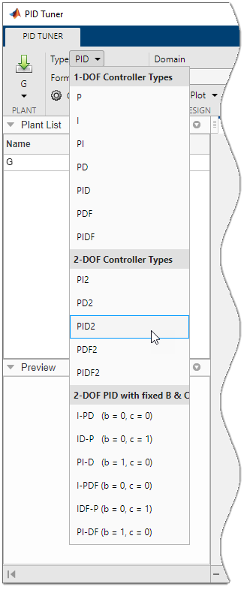## PID Controller Types for Tuning

Control System Toolbox™ PID tuning tools can tune many PID and 2-DOF PID controller types. The term controller type refers to which terms are present in the controller action. For example, a PI controller has only a proportional and an integral term, while a PIDF controller contains proportional, integrator, and filtered derivative terms. This topic summarizes the types of PID controllers available for tuning in the following tools:

### Specifying PID Controller Type

The PID tuning tools let you design numerous controller types. How you specify controller type depends on which tool you are using.

#### Command-Line Tuning

For command-line tuning, provide the `type` argument to the `pidtune` command. For example, ```C = pidtune(G,'PI')``` tunes a PI controller for plant `G`.

Alternatively, if you provide an existing controller object as the input argument `C0`, `pidtune` tunes a new controller of the same type and form. For example, suppose `C0` is a `pid` controller object that has proportional and derivative action only (PD controller). Then, `pidtune(G,C0)` generates a new `pid` controller object that also has only proportional and derivative action. See `pidtune`.

For more about the specific controller types available with command-line tuning, see:

#### PID Tuner App

In the PID Tuner app, you can specify a controller type when you open the app or change controller type within the app.

• Specify type when opening the app — Provide the `type` argument to the `pidTuner` command when you open PID Tuner. For example, `pidTuner(G,'PIDF2')` opens PID Tuner with an initial design that is a 2-DOF PID controller with a filter on the derivative term.

• Specify type with an existing controller object — Provide the baseline-controller `Cbase` argument to the `pidTuner` command when you open PID Tuner. PID Tuner designs a controller of the same type as `Cbase`. For example, suppose `C0` is a `pid` controller object that has proportional and derivative action only (PD controller). Then, `pidTuner(G,C0)` opens PID Tuner with an initial design that is a PD controller.

• Specify controller type within the app — In PID Tuner, use the Type menu to change controller types.For more about the specific controller types available in the PID Tuner app:

#### Tune PID Controller Live Editor Task

In the Tune PID Controller task in the Live Editor, you specify controller type using the Degrees of Freedom and Controller Type menus.For more about the specific controller types available in the Tune PID Controller task, see:

### 1-DOF Controllers

The following table summarizes the 1-DOF PID controller types available with all tools and provides representative controller formulas for parallel form. The standard-form and discrete-time formulas are analogous.

TypeController ActionsContinuous-Time Controller Formula (parallel form)Discrete-Time Controller Formula (parallel form, ForwardEuler integration method)
`P`Proportional onlyKpKp
`I`Integral only

`$\frac{{K}_{i}}{s}$`

`${K}_{i}\frac{{T}_{s}}{z-1}$`

`PI`Proportional and integral

`${K}_{p}+\frac{{K}_{i}}{s}$`

`${K}_{p}+{K}_{i}\frac{{T}_{s}}{z-1}$`

`PD`Proportional and derivative

`${K}_{p}+{K}_{d}s$`

`${K}_{p}+{K}_{d}\frac{z-1}{{T}_{s}}$`

`PDF`Proportional and derivative with first-order filter on derivative term

`${K}_{p}+\frac{{K}_{d}s}{{T}_{f}s+1}$`

`${K}_{p}+{K}_{d}\frac{1}{{T}_{f}+\frac{{T}_{s}}{z-1}}$`

`PID`Proportional, integral, and derivative

`${K}_{p}+\frac{{K}_{i}}{s}+{K}_{d}s$`

`${K}_{p}+{K}_{i}\frac{{T}_{s}}{z-1}+{K}_{d}\frac{z-1}{{T}_{s}}$`

`PIDF`Proportional, integral, and derivative with first-order filter on derivative term

`${K}_{p}+\frac{{K}_{i}}{s}+\frac{{K}_{d}s}{{T}_{f}s+1}$`

`${K}_{p}+{K}_{i}\frac{{T}_{s}}{z-1}+{K}_{d}\frac{1}{{T}_{f}+\frac{{T}_{s}}{z-1}}$`

### 2-DOF Controllers

The tuning tools can automatically design 2-DOF PID controller types with free setpoint weights. The following table summarizes the 2-DOF controller types available in all tools and provides representative controller formulas for parallel form. The standard-form formulas are analogous. For more information about 2-DOF PID controllers generally, see Two-Degree-of-Freedom PID Controllers.

TypeController ActionsContinuous-Time Controller Formula (parallel form)Discrete-Time Controller Formula (parallel form, ForwardEuler integration method)
`PI2`2-DOF proportional and integral

`$u={K}_{p}\left(br-y\right)+\frac{{K}_{i}}{s}\left(r-y\right)$`

`$u={K}_{p}\left(br-y\right)+{K}_{i}\frac{{T}_{s}}{z-1}\left(r-y\right)$`

`PD2`2-DOF proportional and derivative

`$u={K}_{p}\left(br-y\right)+{K}_{d}s\left(cr-y\right)$`

`$u={K}_{p}\left(br-y\right)+{K}_{d}\frac{z-1}{{T}_{s}}\left(cr-y\right)$`

`PDF2`2-DOF proportional and derivative with first-order filter on derivative term

`$u={K}_{p}\left(br-y\right)+{K}_{d}\frac{s}{{T}_{f}s+1}\left(cr-y\right)$`

`$u={K}_{p}\left(br-y\right)+{K}_{d}\frac{1}{{T}_{f}+\frac{{T}_{s}}{z-1}}\left(cr-y\right)$`

`PID2`2-DOF proportional, integral, and derivative

`$u={K}_{p}\left(br-y\right)+\frac{{K}_{i}}{s}\left(r-y\right)+{K}_{d}s\left(cr-y\right)$`

`$u={K}_{p}\left(br-y\right)+{K}_{i}\frac{{T}_{s}}{z-1}\left(r-y\right)+{K}_{d}\frac{z-1}{{T}_{s}}\left(cr-y\right)$`

`PIDF2`2-DOF proportional, integral, and derivative with first-order filter on derivative term

`$u={K}_{p}\left(br-y\right)+\frac{{K}_{i}}{s}\left(r-y\right)+{K}_{d}\frac{s}{{T}_{f}s+1}\left(cr-y\right)$`

`$u={K}_{p}\left(br-y\right)+{K}_{i}\frac{{T}_{s}}{z-1}\left(r-y\right)+{K}_{d}\frac{1}{{T}_{f}+\frac{{T}_{s}}{z-1}}\left(cr-y\right)$`

### 2-DOF Controllers with Fixed Setpoint Weights

With PID control, step changes in the reference signal can cause spikes in the control signal contributed by the proportional and derivative terms. By fixing the setpoint weights of a 2-DOF controller, you can mitigate the influence on the control signal exerted by changes in the reference signal. For example, consider the relationship between the inputs r (setpoint) and y (feedback) and the output u (control signal) of a continuous-time 2-DOF PID controller.

`$u={K}_{p}\left(br-y\right)+\frac{{K}_{i}}{s}\left(r-y\right)+{K}_{d}s\left(cr-y\right)$`

If you set b = 0 and c = 0, then changes in the setpoint r do not feed through directly to either the proportional or the derivative terms in u. The b = 0, c = 0 controller is called an I-PD type controller. I-PD controllers are also useful for improving disturbance rejection.

PID Tuner and `pidtune` can design the fixed-setpoint-weight controller types summarized in the following table. The standard-form and discrete-time formulas are analogous.

TypeController ActionsContinuous-Time Controller Formula (parallel form)Discrete-Time Controller Formula (parallel form, ForwardEuler integration method)
`I-PD`2-DOF PID with b = 0, c = 0

`$u=-{K}_{p}y+\frac{{K}_{i}}{s}\left(r-y\right)-{K}_{d}sy$`

`$u=-{K}_{p}y+{K}_{i}\frac{{T}_{s}}{z-1}\left(r-y\right)-{K}_{d}\frac{z-1}{{T}_{s}}y$`

`I-PDF`2-DOF PIDF with b = 0, c = 0

`$u=-{K}_{p}y+\frac{{K}_{i}}{s}\left(r-y\right)-{K}_{d}\frac{s}{{T}_{f}s+1}y$`

`$u=-{K}_{p}y+{K}_{i}\frac{{T}_{s}}{z-1}\left(r-y\right)-{K}_{d}\frac{1}{{T}_{f}+\frac{{T}_{s}}{z-1}}y$`

`ID-P`2-DOF PID with b = 0, c = 1

`$u=-{K}_{p}y+\frac{{K}_{i}}{s}\left(r-y\right)+{K}_{d}s\left(r-y\right)$`

`$u=-{K}_{p}y+{K}_{i}\frac{{T}_{s}}{z-1}\left(r-y\right)+{K}_{d}\frac{z-1}{{T}_{s}}\left(r-y\right)$`

`IDF-P`2-DOF PIDF with b = 0, c = 1

`$u=-{K}_{p}y+\frac{{K}_{i}}{s}\left(r-y\right)+{K}_{d}\frac{s}{{T}_{f}s+1}\left(r-y\right)$`

`$u=-{K}_{p}y+{K}_{i}\frac{{T}_{s}}{z-1}\left(r-y\right)+{K}_{d}\frac{1}{{T}_{f}+\frac{{T}_{s}}{z-1}}\left(r-y\right)$`

`PI-D`2-DOF PID with b = 1, c = 0

`$u={K}_{p}\left(r-y\right)+\frac{{K}_{i}}{s}\left(r-y\right)-{K}_{d}sy$`

`$u={K}_{p}\left(r-y\right)+{K}_{i}\frac{{T}_{s}}{z-1}\left(r-y\right)-{K}_{d}\frac{z-1}{{T}_{s}}y$`

`PI-DF`2-DOF PIDF with b = 1, c = 0

`$u={K}_{p}\left(r-y\right)+\frac{{K}_{i}}{s}\left(r-y\right)-{K}_{d}\frac{s}{{T}_{f}s+1}y$`

`$u={K}_{p}\left(r-y\right)+{K}_{i}\frac{{T}_{s}}{z-1}\left(r-y\right)-{K}_{d}\frac{1}{{T}_{f}+\frac{{T}_{s}}{z-1}}y$`